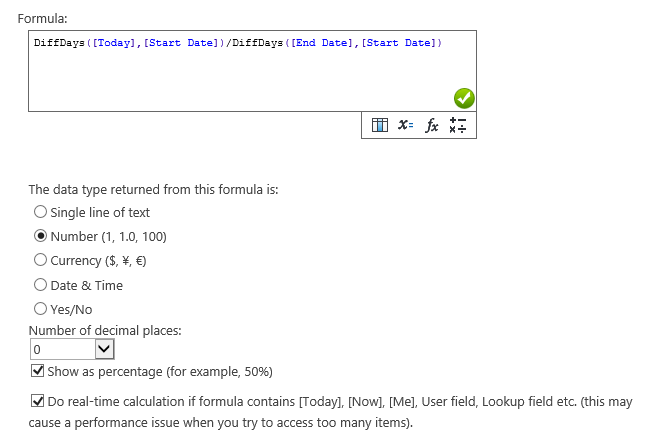# Calculated Boost Field Use Cases

Calculated Boost Field is a field which enables you to do calculate based on other columns like SharePoint Calculated column. With Calculated Boost, you can design powerful formula and complete complicated calculation.

Calculated Boost supports more column types than SharePoint OOTB calculated column, including People, Lookup, Note, ID, Content Type etc. Even better, you can use [Today], [Me] and [Now] variables in the formula.

The following cases will demonstrate how to use Calculated Boost Field.

[Case 1]

Case list records the cases status, and there is a date field “Start date” in the list. I want to create field based on below condition.

If end date is less than current date enter value “Done”.

If end date is greater than current date enter value “Doing”.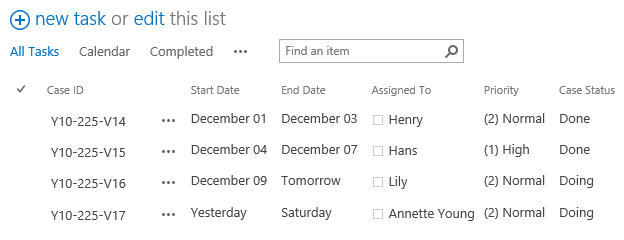In the Calcuated Boost field, you can design the formula as this:

if([End Date]<=[Today],”Done”,”Doing”)[Case 2]

I have a Subscribers list which is recording the email addresses, and I want to extract the user name from the address. The email in column is like hans@gmail.com, the user name should be hans.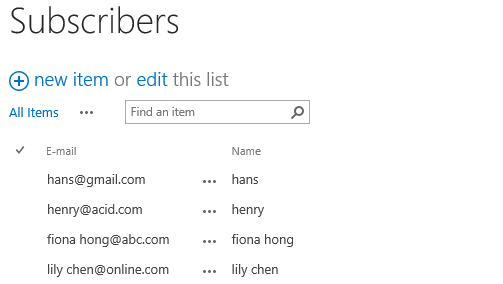In the Calcuated Boost field, design the formula as this:

SubString([E-mail], 0, IndexOf([E-mail],”@”))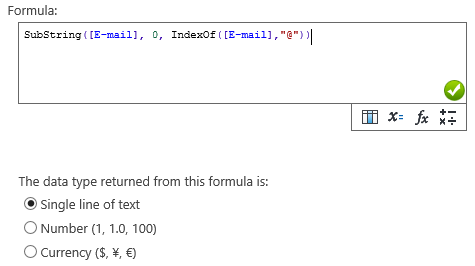[Case 3]

I have a project list which is recording the project status. I want to create a column to show percentage completed based on the End Date, current date and Start Date.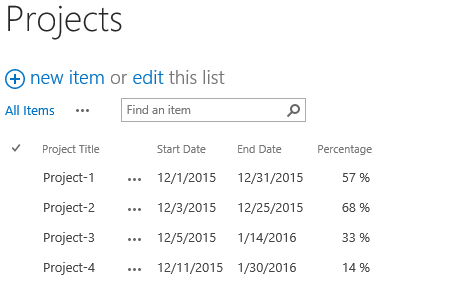In the Calcuated Boost field, design the formula as this:

DiffDays([Today],[Start Date])/DiffDays([End Date],[Start Date])